Fractions - math word problems - page 14

1. Pie II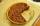Vili ate three pieces of pie. If it pieces is 1/8 how much pie did he eat?
2. PearsThere were pears in the basket, I took two-fifths of them, and left six in the basket. How many pears did I take?
3. Percentages 5\$5.25 is 7 1/2% of what number?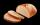Several pieces of bread were in the store. Half of them plus a half of bread bought a cookbook. Of the remaining bread, half of it plus half of the bread was bought by Mr. Novák. The last bread was bought by Ms. Small. How many breads were in the store at.
5. Unit rateFind unit rate: 6,840 customers in 45 days
6. PercentagesWhat is 1/3 of \$821.43?
7. Equation with xSolve the following equation: 2x- (8x + 1) - (x + 2) / 5 = 9
8. Two brothersThe two brothers were to be divided according to the will of land at an area of 1ha 86a 30m2 in a ratio of 5:4. How many will everyone get?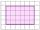Shade the area on the grid that shows 5/8 x 2/4
10. Image reduction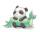To say that I am mathematically challenged is a gross understatement! I'm trying to help my friend determine how to calculate percentages of reduction or enlargement for a quilt pattern. She is creating a drawing of a cute picture which will become a pie
11. Hamster cage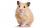Ryan keeps his hamster cage on his dresser. The area of the top of Ryan's dresser is 1 2\3 as large as the area of the bottom of his hamster cage. The area of the dresser top is 960 square inches. How many square inches of his dresser top are not covered b
12. Simple equation 324 = n • 27, solve for n
13. Land areaA land area of Asia and Africa are in a 3: 2 ratio, the European and African are is 1:3. What are the proportions of Asia, Africa, and Europe?
14. Evaluate expressionEvaluate expression using BODMAS rule: 1 1/4+1 1/5÷3/5-5/8
15. Stones in aquariumIn an aquarium with a length of 2 m, 1.5 m wide and 2.5 m deep, the water is up to three-quarters of the depth. Can we place 2m cubic meters of stones in the aquarium without spilling water? (0 = no, 1 = yes)
16. The thirdThe one-third rod is blue, one-half of the rod is red, the rest of the rod is white and measures 8 cm. How long is the whole rod?
17. 3 children3 children had to divide 4 pounds is candy. How much candy did each child get?
18. Cube, cuboid, and sphereVolumes of a cube and a cuboid are in ratio 3: 2. Volumes of sphere and cuboid are in ratio 1: 3. In what ratio are the volumes of cube, cuboid, and sphere?
19. Interior designer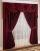To make draperies an interior designer needs 11 1/4 yards of material for the den and 8 1/2 yards for the living room. If material comes only in 20 yard bolts, how much will be left over after completing both sets of draperies?
20. TogetherGrandfather, father, and son are carpenters. They decided to make a wardrobe. Father produces it for 12 hours, his son for 15 hours, and grandfather for 10 hours. How long will they make it together?

Do you have an interesting mathematical word problem that you can't solve it? Enter it, and we can try to solve it.

To this e-mail address, we will reply solution; solved examples are also published here. Please enter e-mail correctly and check whether you don't have a full mailbox.

Need help calculate sum, simplify or multiply fractions? Try our fraction calculator.